We will now discuss the icosahedron and its associated Archimedean and Catalan solids.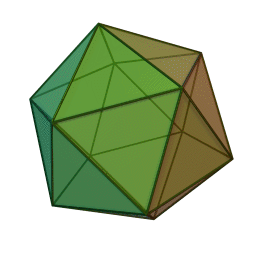The Icosahedron – 3600°

The icosahedron is the shape that gives the most symmetrical distribution of points, edges, and surfaces on the sphere.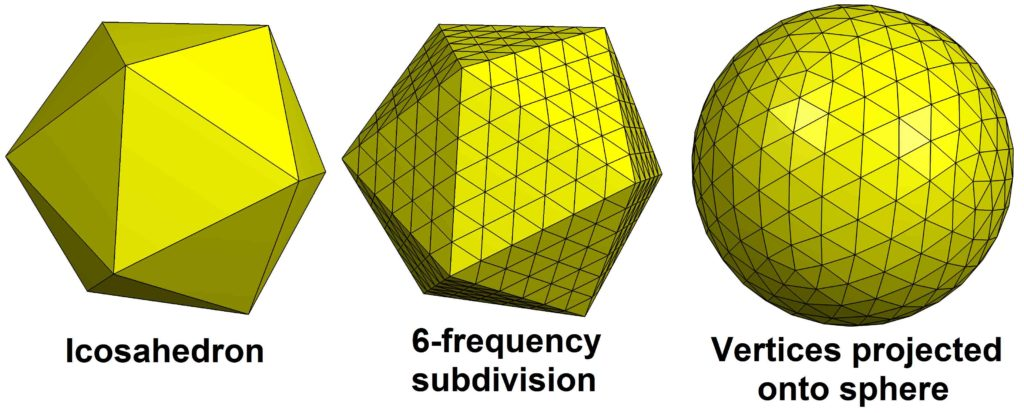It has:

• 20 Faces (20 equilateral triangles) 5 to a vertex
• 30 edges
• 12 corners

It’s Dual is the dodecahedron.If the icosahedron has edge length = 1,

the dual dodecahedron will have edge length = (√5 – 1)/2  or 1/phi.

The icosahedron is associated with ‘Water’.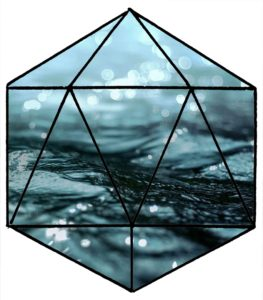To Plato, water was the densest and least penetrating of the three fluid elements: fire, water and air.

In modern times the large-scale clustering of tetrahedral water molecules have been found to take the shape of an icosahedron.   We discuss this in detail in Article 173.

The Net of the Icosahedron

An icosahedron has 43,380 distinct nets.  These are all the ways 20 equilateral triangles can be arranged to fold into the icosahedron.

Here is one: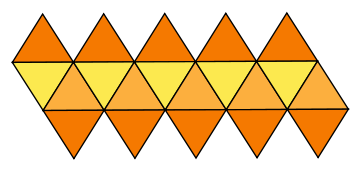The Icosahedron has:

• 15 2-fold axes
• 10 3-fold axes
• Six 5-fold axes

Projections of the Icosahedron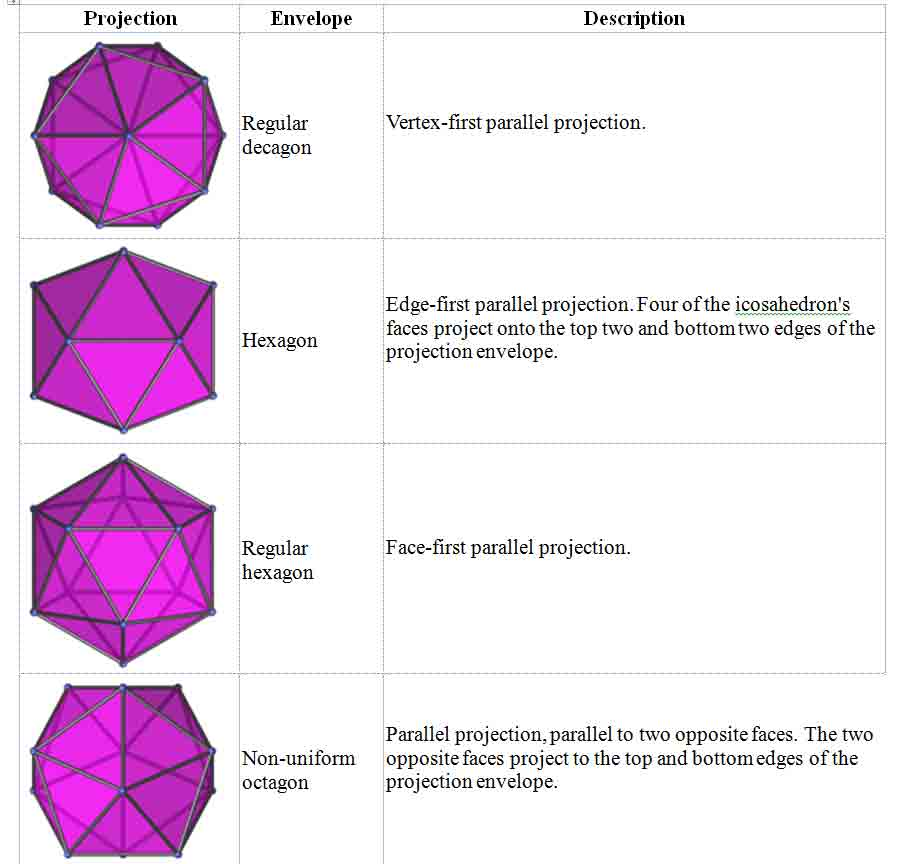Spherical Close-Packing

The Icosahedron – 12 spheres – This grouping without the nucleus (as in the cuboctahedron) closes into the triangulation of the icosahedral grouping.  Each sphere touches five others.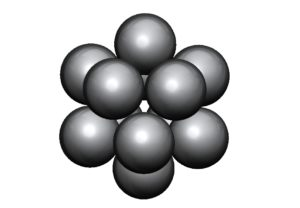Arranging twelve equal spheres to define an icosahedron (as seen above) leaves space at the center for another sphere just over 9/10ths as wide as the others.

The icosahedron (and its dual the dodecahedron) can also be made from 32 close-packed spheres.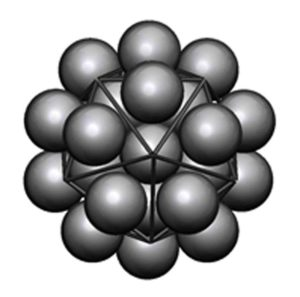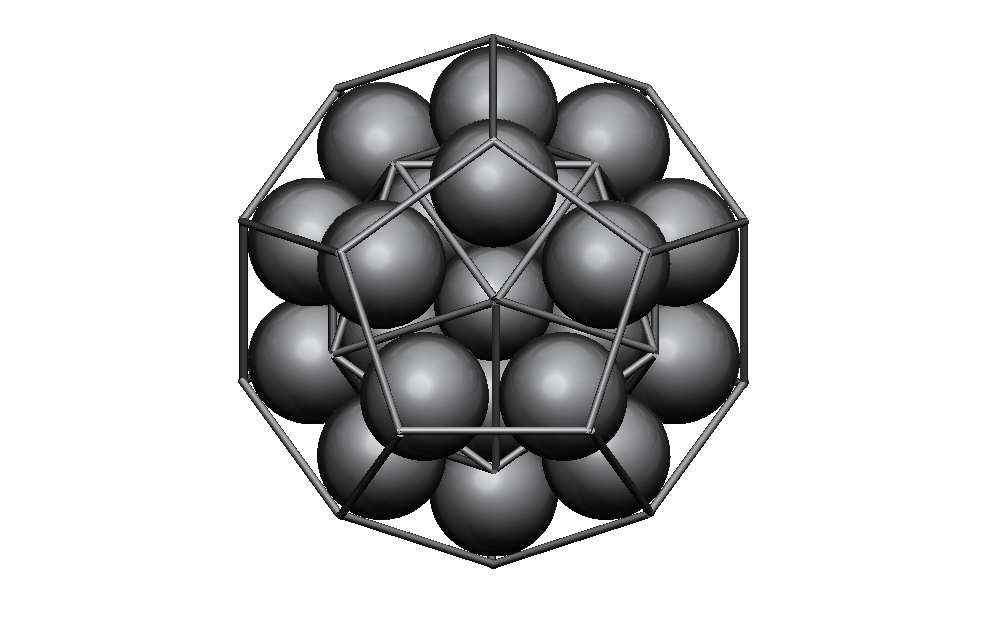Here is the Icosahedron in Metatron’s Cube: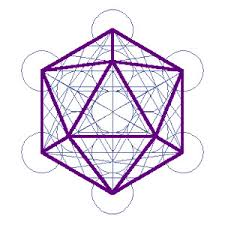The Spherical Icosahedron: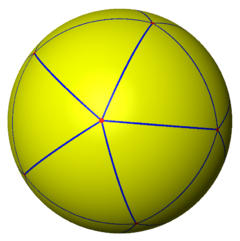Areas and Volume of the Icosahedron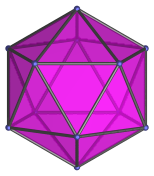Area = 5√3s2              or                     5√3s2                           s = side length

Volume = 5/12 (3 + √5)s3       or         5/6 φ2s3                        s = side length

3600°

The Sum of the Icosahedron’s Angles = 3600º.

There are:

• 360 degrees in a circle
• 360° = sum of angles of a square (90° x 4)
• 3600° = sum of angles of an icosahedron

3600 hertz = A#

The Sum of the angles of the first four solids that correspond to the Four Elements: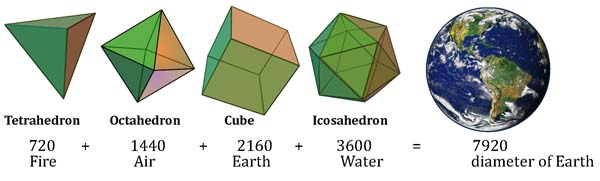Tetrahedron (fire) 720 + Octahedron (Air) 1440 + Cube (Earth) 2160 + Icosahedron (Water) 3600 = 7920 = diameter of the Earth in miles (99.97% accuracy).

8 x 9 x 10 x 11 = 7920

Phi in the Icosahedron

The 12 vertices of the icosahedron are defined by 3 perpendicular Golden Section rectangles.  These are seen below in dark green, light green and purple.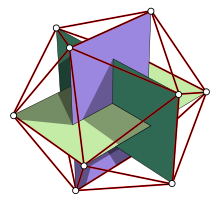A rectangle drawn inside an icosahedron has edges in the ratio φ:1 (or 1:φ).

Nested inside an octahedron, the icosahedron cuts its edges in the ratio φ:1.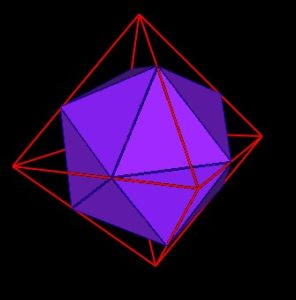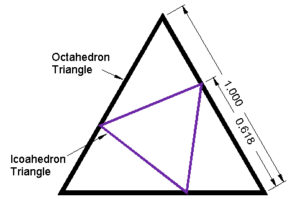The truncated icosahedron (common soccer ball or C60) contains a rectangle with edges in the ratio 3φ:1.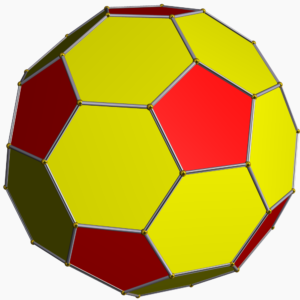The icosidodecahedron has a radius:edge of φ:1.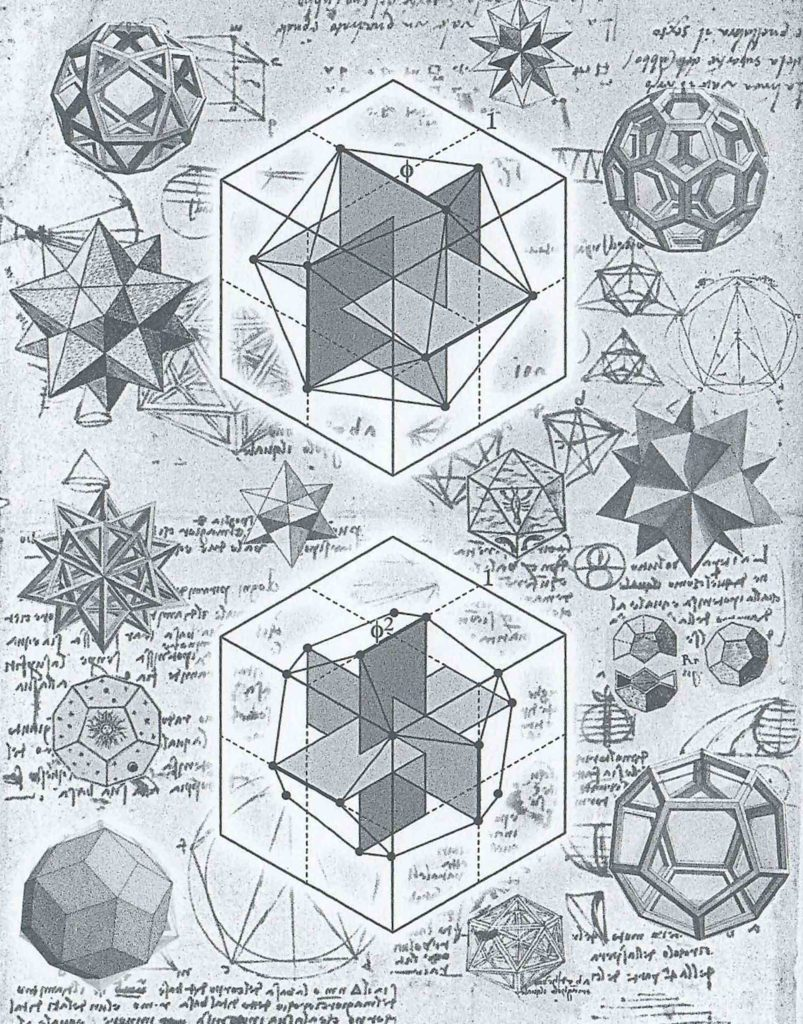Credit: page 341 in Designa.

The Icosahedron is made of equilateral triangles arranged into five-sided pentagonal shapes called icosahedral caps.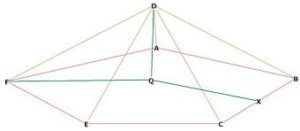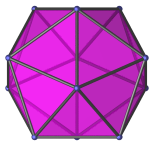As Buckminster Fuller taught, the icosahedron ‘dimples’ easily.  “Push hard on one vertex and five triangles cave in, such that the tip of the inverted pyramid reaches just beyond the icosahedron’s center of gravity.”1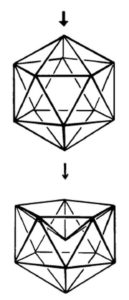The Icosahedron in Chemistry

In chemistry, in the work of Dr. Robert Moon, the icosahedron is the element Iron.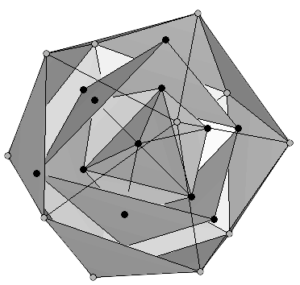Start with the cube: 8 corners = 8 protons.  Element = oxygen.

Next is octahedron: (8 points of the cube + 6 points of the octahedron = 14) 14 points = 14 protons.  Element = silicon.

Third is the icosahedron: (12 corners + 14) 26 corners = 26 protons.  Element = Iron.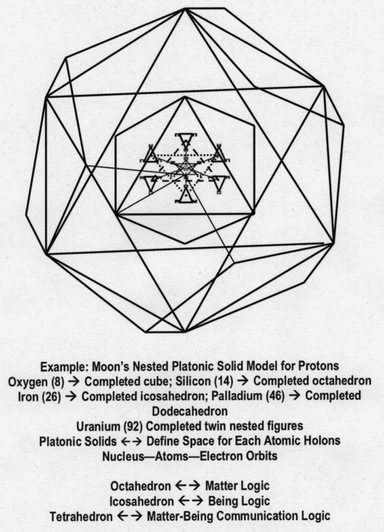The Icosahedron and Water

In the mineral world, the icosahedron is associated with large-scale clustering of water.

Plato corresponded water to the icosahedron.  “Water, the icosahedron, flows out of one’s hand when picked up, as if it is made of tiny little balls.”2

A single molecule (H20) is tetrahedral in nature.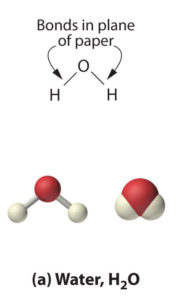The work of Martin Chaplin shows us that tetrahedral water molecules form icosahedral clusters, linking modern science with ancient philosophy.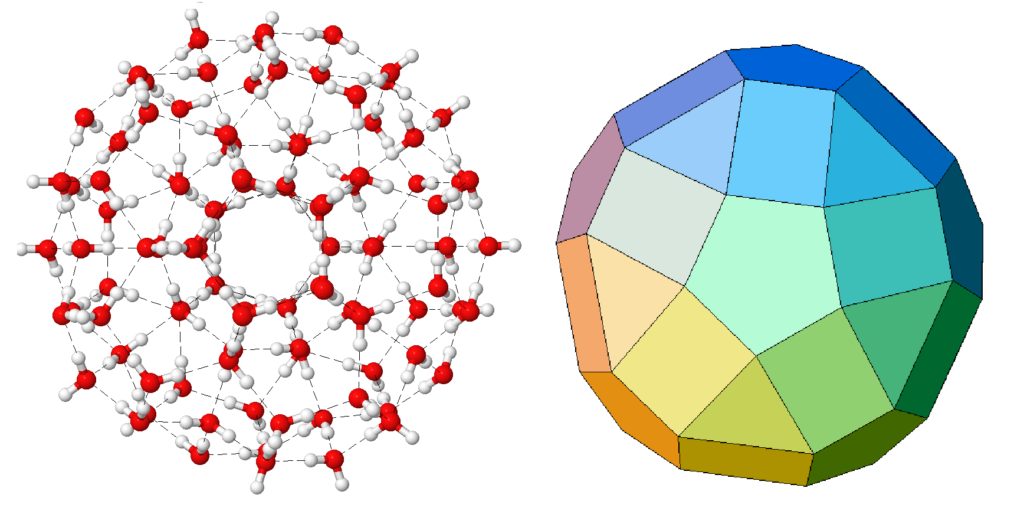In its liquid form water has a tendency to create pentagonal geometry; when it is frozen it goes from constantly shifting pentagonal formation to a hexagonal crystal array.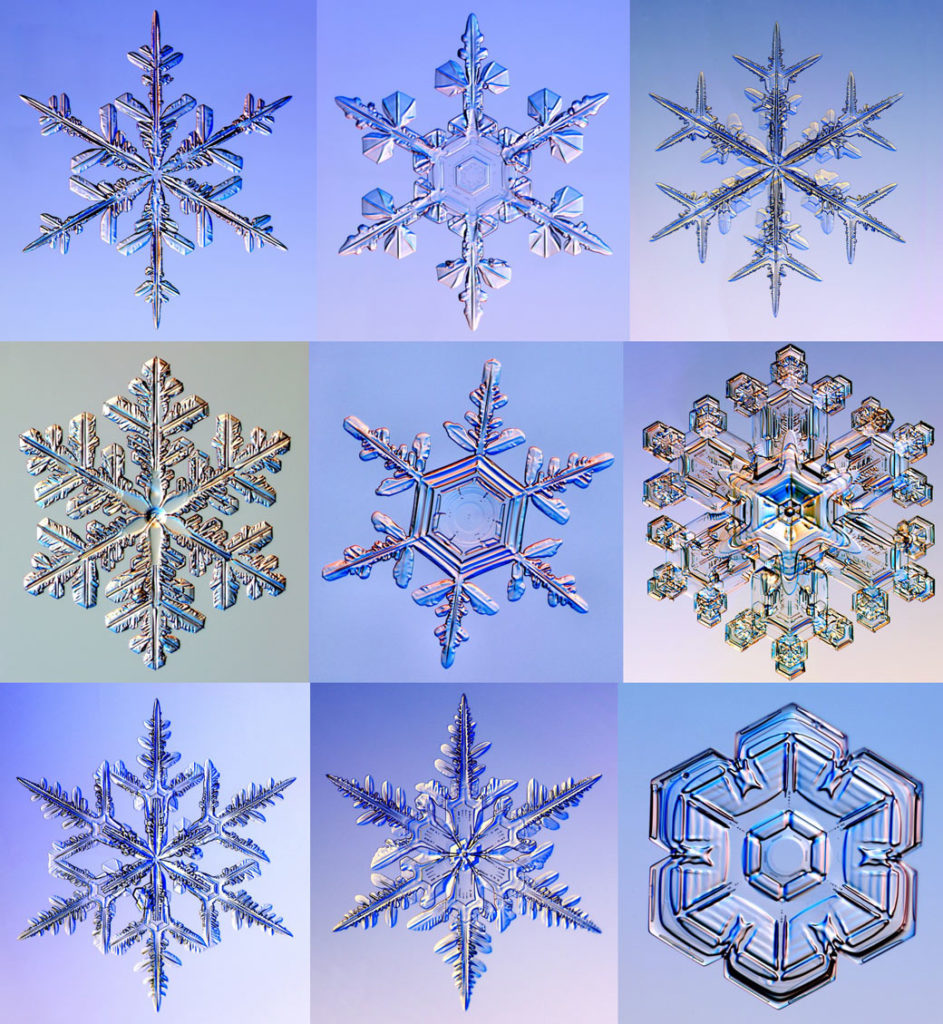Chaplin tells us, “The golden ratio occurs in the dimensions of the pentamers of water molecules that are commonly found in liquid water and water icosahedra.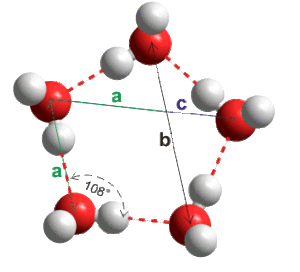“The water lattice begins as 4-fold, tetrahedral units of 14 water molecules, aligning into 20 clusters to create the geometry of a 280-molecule, water icosahedron. This structure then assumes a variety of stable, geometric sub-structures, such as its complementary, opposite dodecahedron, that form into even larger ‘superclusters’…There is nothing random or arbitrary about this – it is an inevitable outcome of the physics of harmonics acting at the atomic level.”3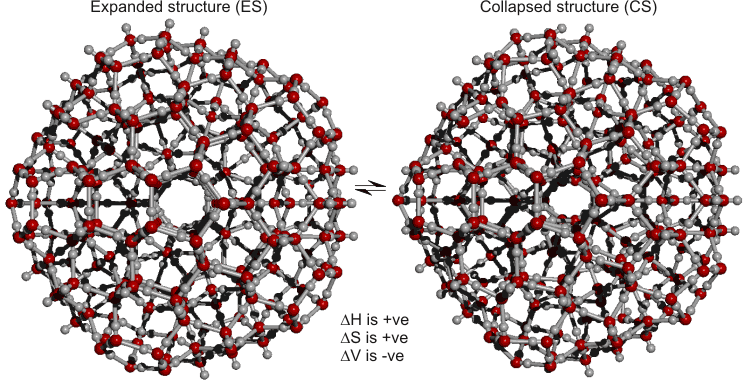Many borides and allotropes of boron contain boron B12 as a basic structure unit.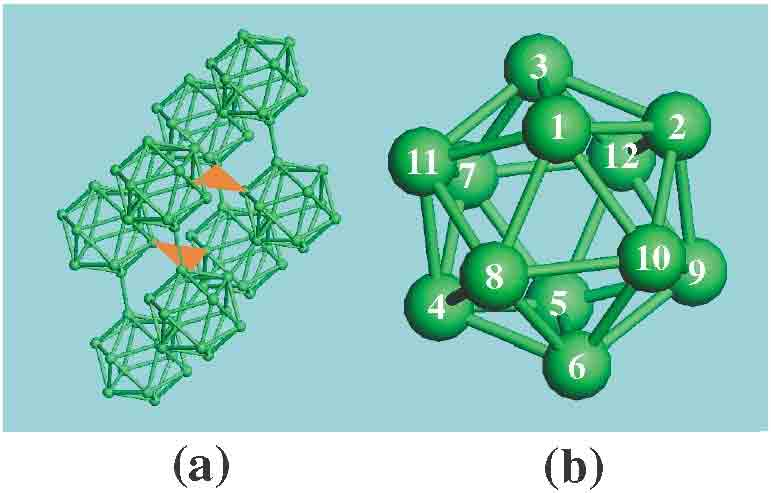The Icosahedron in Nature

The closo-carboranes are chemical compounds with shape very close to an icosahedron.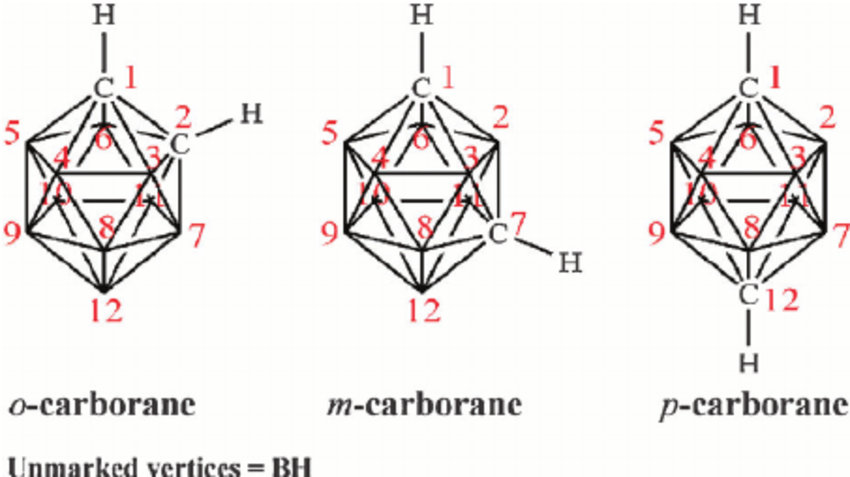Icosahedral twinning also occurs in crystals, especially nanoparticles.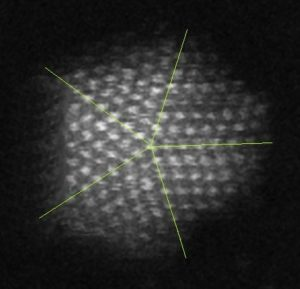Transmission electron microscopy image of a 5-fold twinned icosahedral gold (Au) nanoparticle.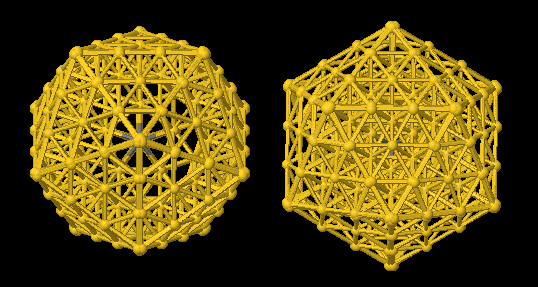FCC icotwin model projected down 5-fold & 3-fold zone axis orientations.  Credit:  P. FraundorfOwn work

Quasicrystals are icosahedral (and dodecahedral) in structure.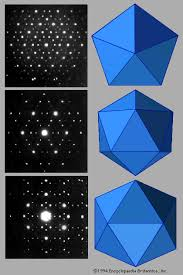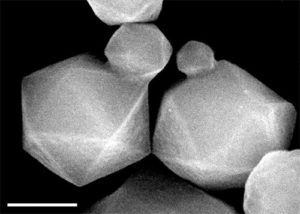The icosahedron shows up in the structure of many viruses including measles, herpes and HIV.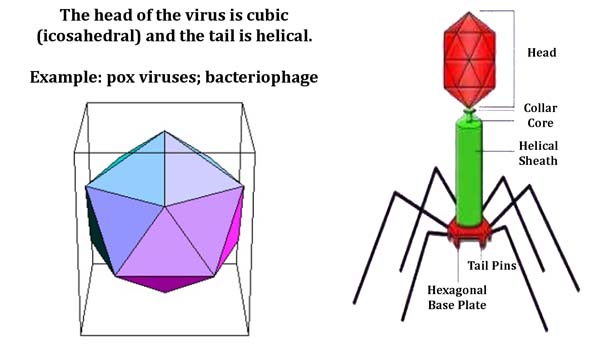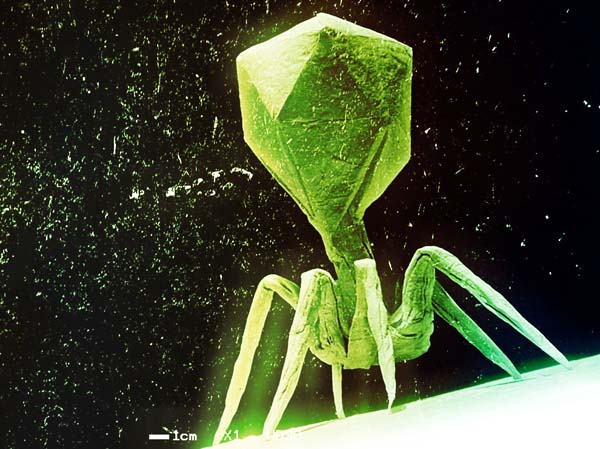Bacteriophage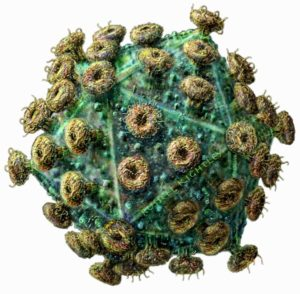Measles Virus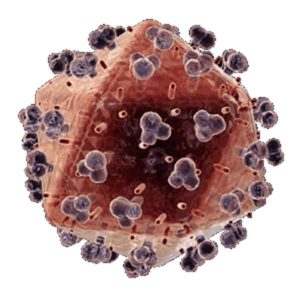HIV Virus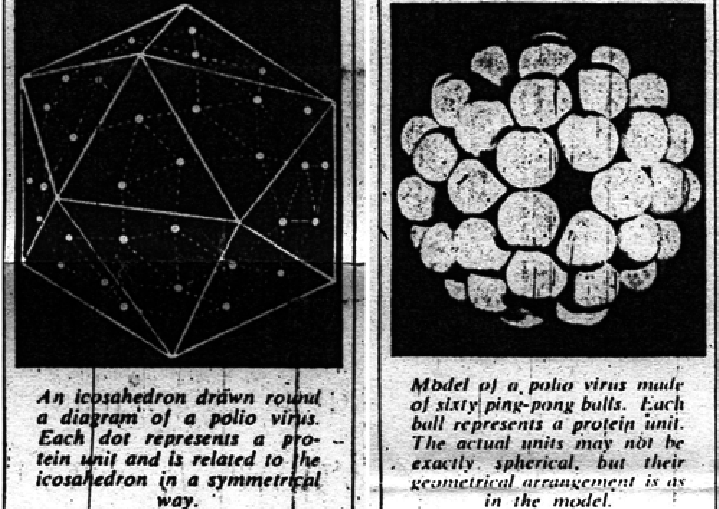Polio Virus – printed in The Observer June 21, 1959

The icosahedron shows up in the shape of many radiolaria (zooplanckton).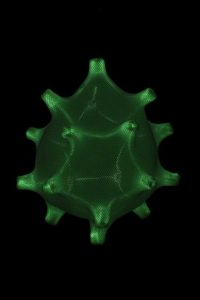Tentaculus Giganticus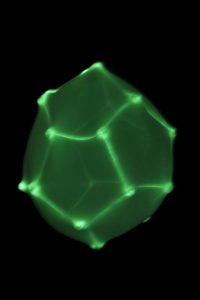Pseudoglobulus footballi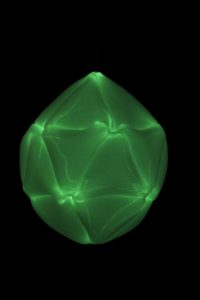Metamorphosus Lucidus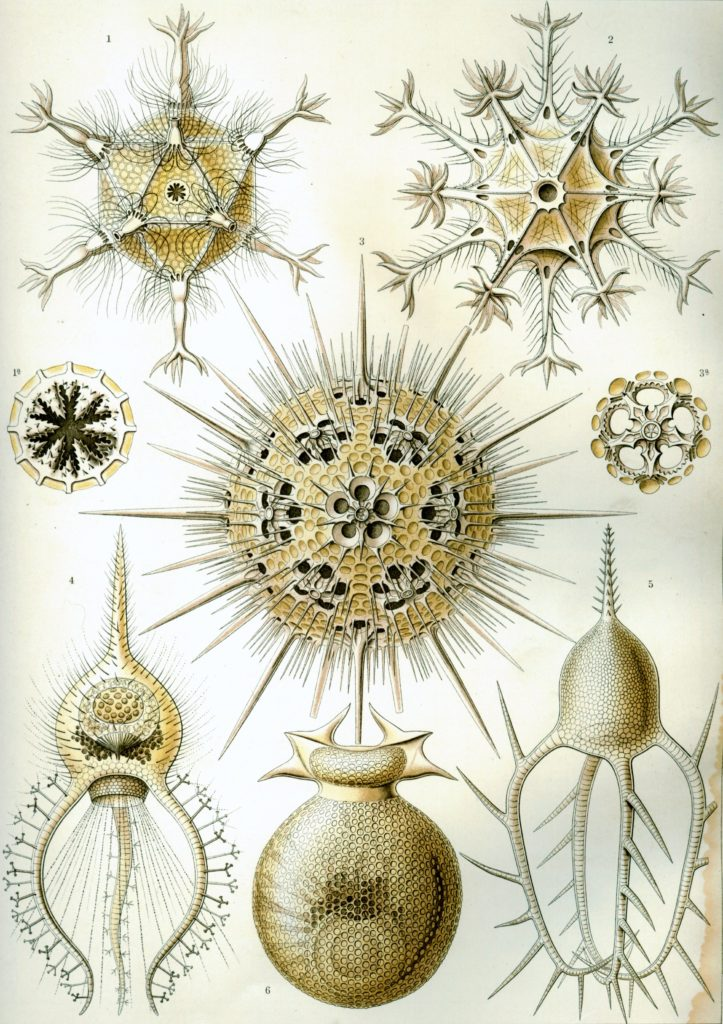Plate 1: Phaeodaria – Ernst Haeckel – Art Forms in Nature

Icosahedral structuring also shows up in the shape of many pollen grains.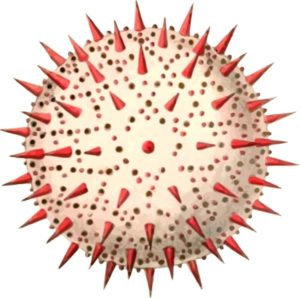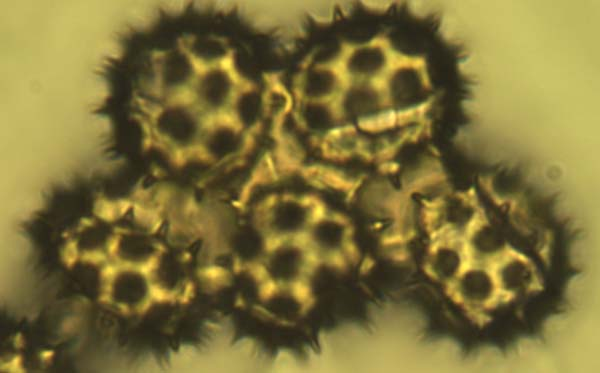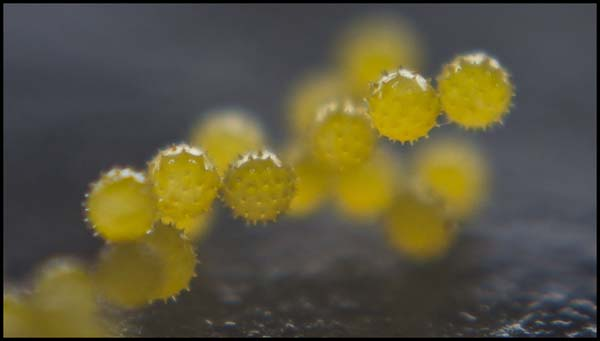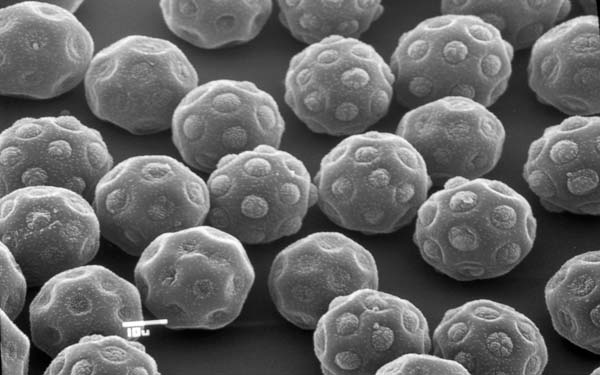The icosahedron also shows up in the global grid or “matrix of cosmic energy”.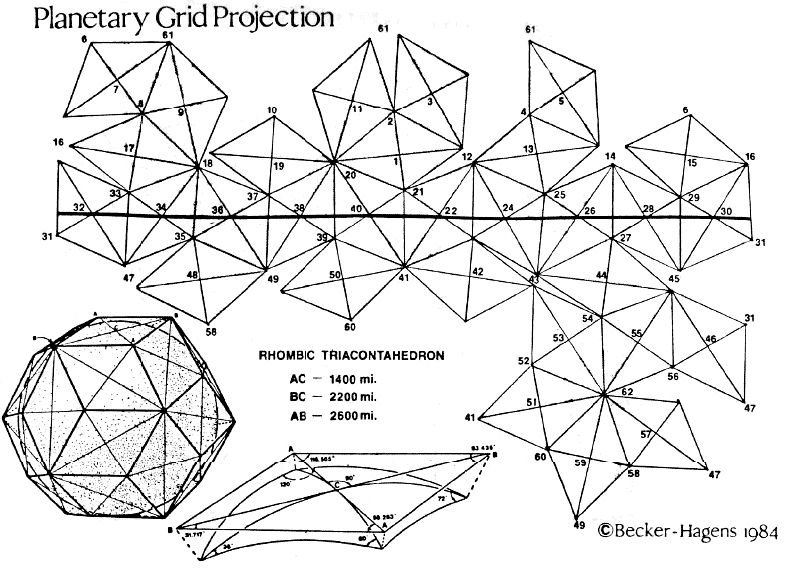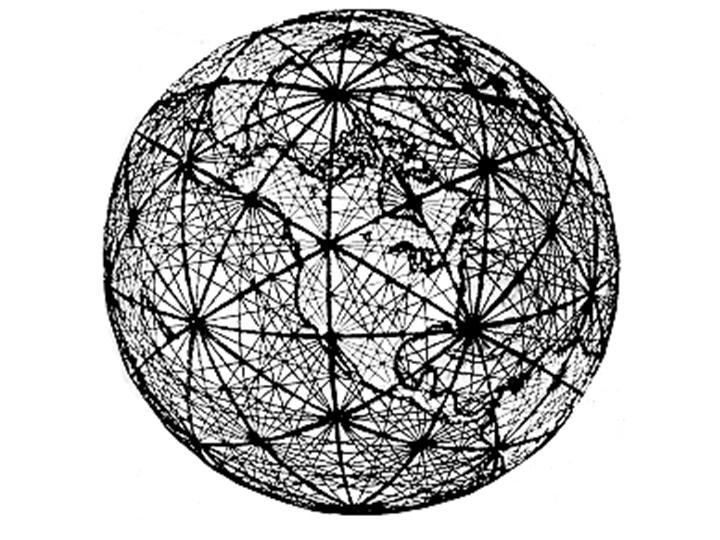Many of the greatest earthquake-prone seismic fracture lines were directly on the grid.

Undersea volcanic mountain ridges often lined up perfectly, as well as above-ground mountain ranges.

Some of these include “the bigger part of the Mid-Atlantic range, Lomonosov ridge in the Arctic Ocean, underwater ranged around Antarctica, an area of the Owen Break in the Indian Ocean, an Anchorage Pradho-Bay Break in Alaska, and a giant break from Morocco to Pakistan.”3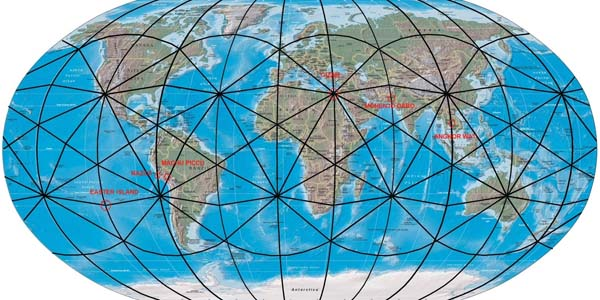Higher and lower pressure zones also appeared in some of the areas where any three lines crossed – of which there were 62 places.

These vortex areas formed the center points for major ocean currents and whirlpools.

The areas of highest and lowest geomagnetic Gauss strength fit neatly within this geometry.

Significant concentrations of ores and petroleum appeared in these areas.

“Iron, nickel and copper tend to concentrate near dodecahedron ribs and nodes, while oil, uranium, and diamonds tend to concentrate near icosahedron ribs and nodes.”4

All of these scientific concepts are discussed in detail throughout the Science section of Cosmic Core.

The Geodesic Dome

The shape of the Geodesic Dome was discovered by Buckminster Fuller.It is formed by subdividing the faces of an icosahedron into smaller triangles then projecting the new vertices outward to the same distance from the center as the original ones.It is used in architecture.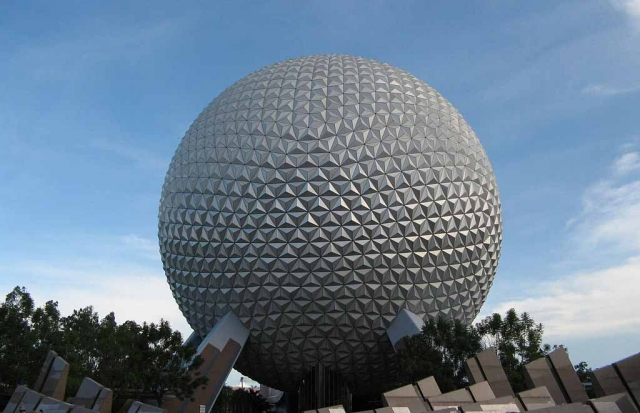Fuller also created the “Dymaxion map” or “Fuller map”.  It is a projection of a world map onto the surface of an icosahedron.  Its maximum distortion is only 2%.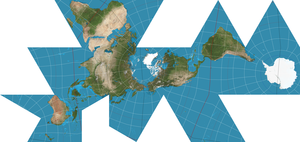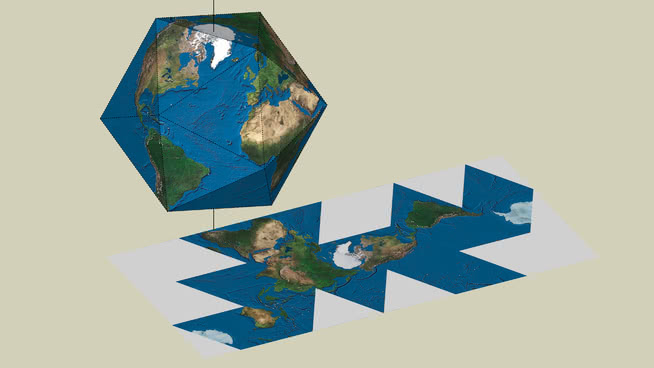Rudolf Laban & the Icosahedron

Rudolf Laban, a famous dance teacher, found that the maximum angles through which human limbs move are identical to those angles of the 20-sided icosahedron.

He created dances whose architecture in time was identical with spatial harmony of crystals.

If a dancer had small lights on his or her limbs and danced in a dark room, the different Platonic solids could be traced by their movements.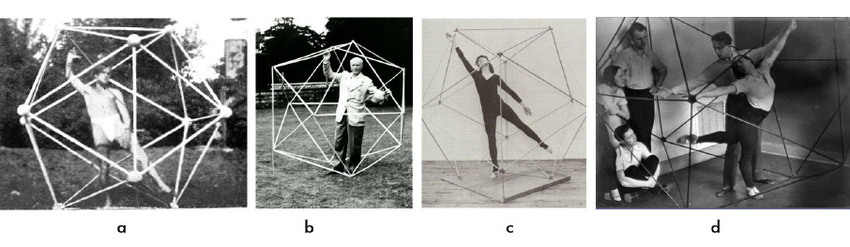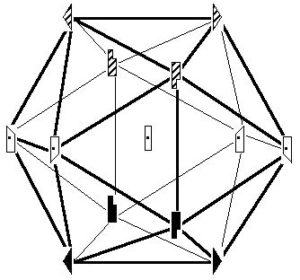Note here that Pentagon/Phi geometry is used to project and share, in contrast to Cubic/Hexad/Octad geometry which is used to hold, make membranes, contain and create barriers such as in the structure of cells.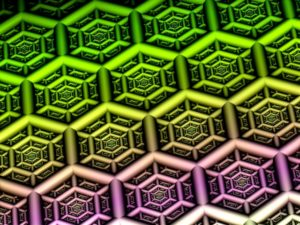In other words, cubic, hexad and octad geometry is used to create barriers.  Pentagon and phi geometry is used for breaking through barriers.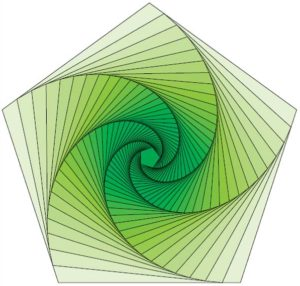Tetrahedron and Icosahedron

Note – it takes 20 tetrahedrons to form an icosahedron.  Each face of the icosahedron would be connected to the centroid (center point of the icosahedron).

However, the tetrahedrons would have to be compressed somewhat to form a perfect icosahedron.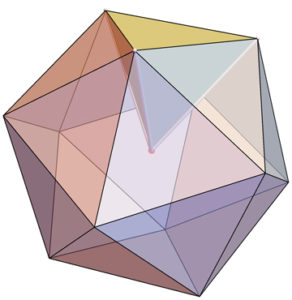If each icosahedron edge is 1 unit long, then the tetrahedron edges incident to the centroid (center point of the icosahedron) have length:

½ [√½ (5+√5)] ≈ 0.95

The tetrahedra would be slightly non-regular when constructed in this way.5

Furthermore, the compound of ten tetrahedra, mentioned below, is one of the stellations of the icosahedron.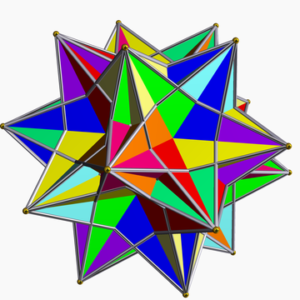The Icosahedron & Octahedron

An icosahedron nests within an octahedron.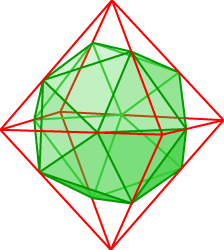Each of the 12 edges of the octahedron correspond to the 12 vertices of the icosahedron.

The icosahedron’s vertices cut the octahedron’s edges perfectly in the Golden Section.It is interesting to note here that the octahedron and icosahedron are different phases of the Jitterbug motion.  Additionally, the 8 triangles of the Jitterbug pass through a regular dodecahedron phase.

“If the position of one of the Jitterbug triangles in the dodecahedron position in drawn in the triangular face of an octahedron, we get the following diagram:”4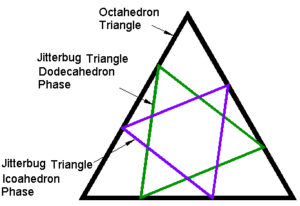Furthermore, by angularly tilting the octahedron on its side (we have not calculated the exact number of degrees of tilt that are necessary,) and adding a special harmonic tetrahedral shape in twelve different places, we can build the icosahedron.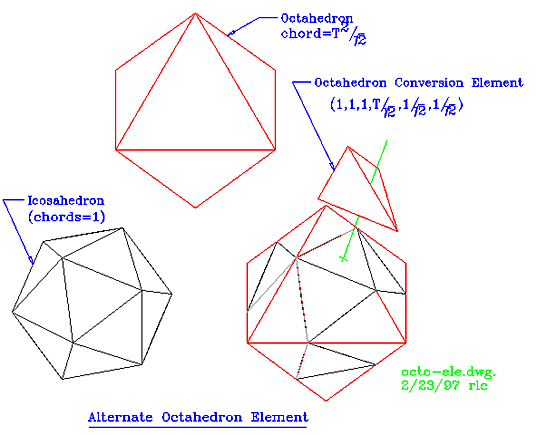Credit: David Wilcock

The Icosahedron & Dodecahedron

The Icosahedron and dodecahedron are duals.  Connecting the centers of the faces creates the dual.Or, the dodecahedron’s points can be truncated to yield the icosahedron.The below diagram, created on Bruce Rawle’s Sacred Geometry website shows the Platonic solids interpenetrating with their duals.  The dodecahedron into an icosahedron is shown on the far right.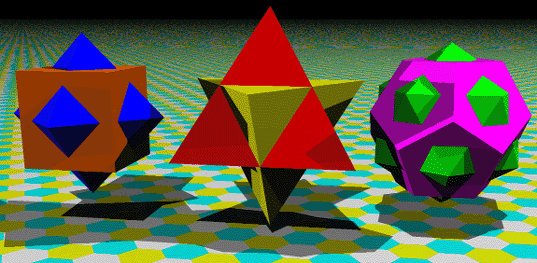Stellations of the Icosahedron

There are 58 stellations of the icosahedron.

These include 1 Kepler-Poinsot solid, 4 polyhedron compounds, and 1 dual polyhedron of an Archimedean solid.

One of the stellations of the icosahedron is the compound of ten tetrahedra.32 of the stellations have full icosahedral symmetry.

27 are enantiomeric forms.  This means they are mirror images of other stellations.

The chart below shows all stellations including the original icosahedron (#1).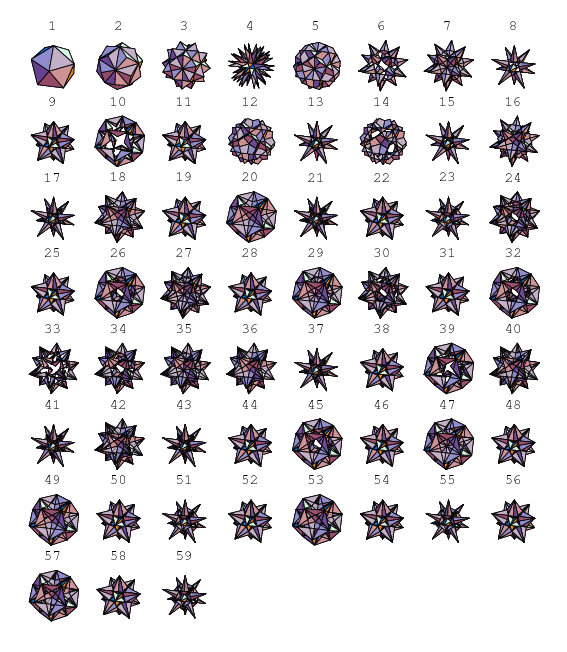The Truncated Icosahedron – 20800º

We will now discuss the Archimedean solid, the Truncated Icosahedron.It has:

• 32 Faces (12 regular pentagonal; 20 regular hexagonal)
• 60 Vertices
• 90 Edges

The Sum of its Angles = 20800°.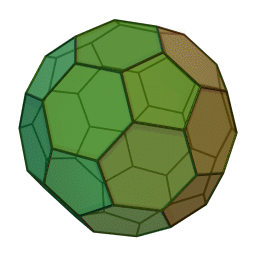Projections of the Truncated Icosahedron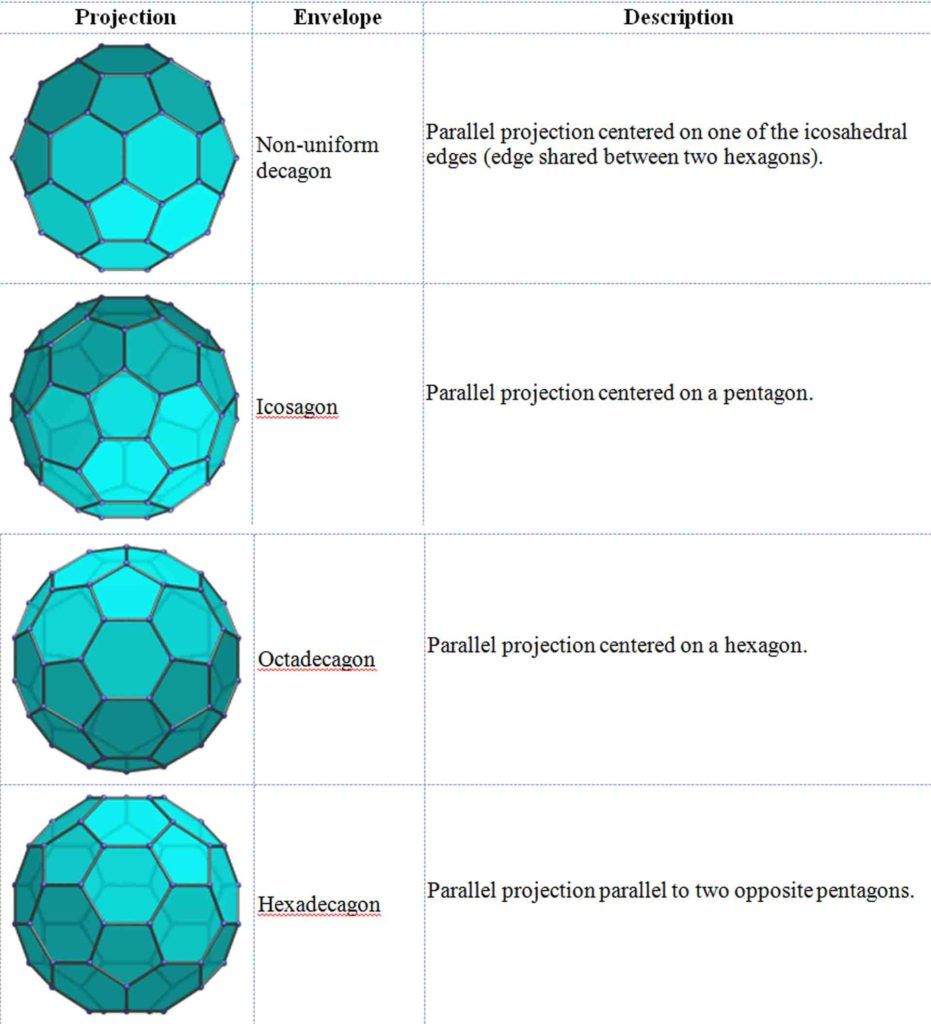The Spherical Truncated Icosahedron: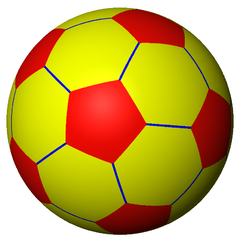Note that this is the exact pattern of a soccer ball.

Net of the Truncated Icosahedron: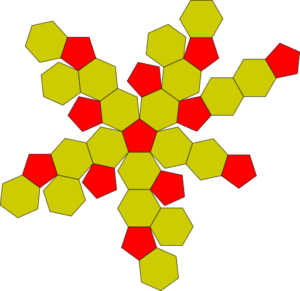The Truncated Icosahedron is also the shape of the Fullerene molecule (Buckyball), an allotrope of carbon, discovered in 1985.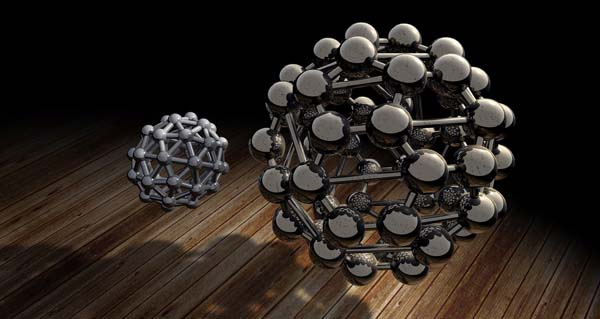Pentakis Dodecahedron – 10800º

The dual of the Truncated Icosahedron is the Catalan solid, the Pentakis Dodecahedron.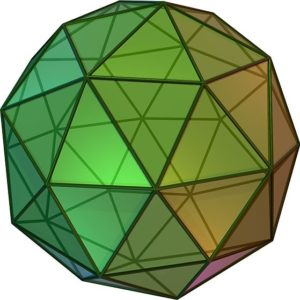It has:

• 60 isosceles triangle faces
• 90 edges
• 32 vertices

The faces look as follows: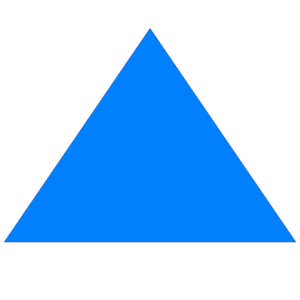The sum of its angles = 10800°.

The Net of the Pentakis Dodecahedron: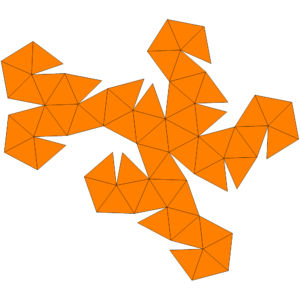Projections of the Pentakis Dodecahedron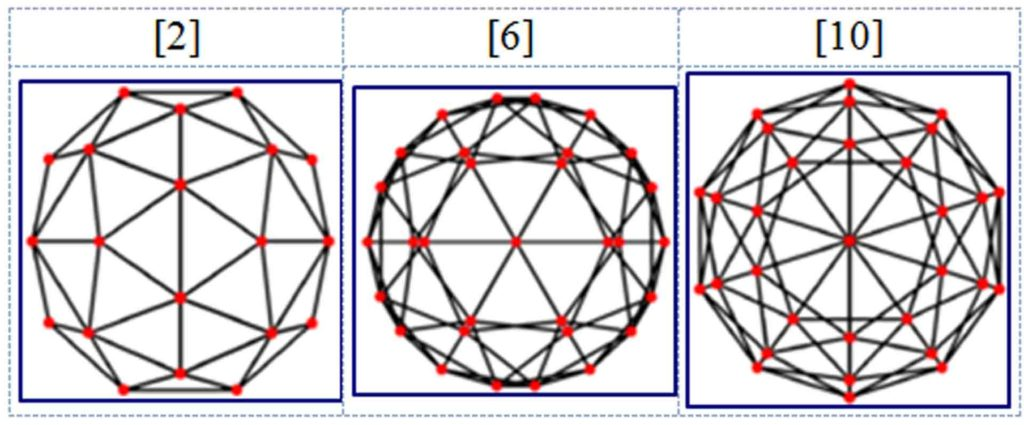The Spherical Pentakis Dodecahedron: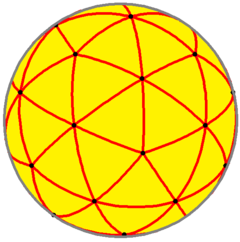The pentakis dodecahedron can be used as a model of the fullerene molecule, as well as the truncated icosahedron.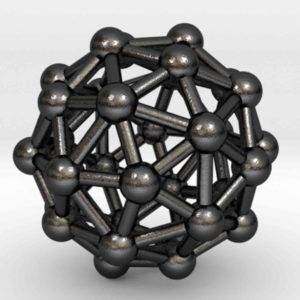The pentakis dodecahedron can also model certain viruses such as the Adeno-associated virus.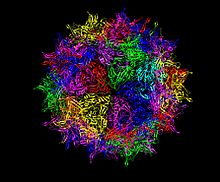1. Edmondson, Amy, A Fuller Explanation: The Synergetic Geometry of R. Buckminster Fuller, Burkhauser Boston, 1987
2. Lawlor, Robert, Sacred Geometry: Philosophy & Practice, Thames & Hudson, 1984
3. https://sapientiaexanimo.wordpress.com/2015/02/19/the-universal-harmonic-pattern-of-life/#more-449
4. Kovalenko, Kostya, A Russian Theory of the Energy Grids, http://www.spiritofmaat.com/archive/nov2/thegrids.htm
5. https://math.stackexchange.com/questions/1340470/how-to-make-an-icosahedron-from-20-tetrahedra